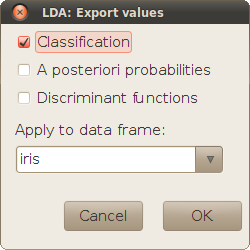## Description

Linear Discriminant Analysis (LDA) is a statistical technique used to investigate the relation between a set of continuous, normally distributed independent variables and a categorical outcome. This objective is similar to binary or multinomial logistic regression, although the calculation procedures and assumptions about the data are different. LDA follows a Bayesian approach, that defines an a priori classification just based on frequencies, and then adjusts it according to the relations observed between variables.

LDA defines as many 'discriminant functions' as the number of categories of the outcome minus one, so that each function is a linear combination of the independent variables. As in Principal Component Analysis or Factor Analysis, these functions are ordered by the amount of variance that the explain, and if the number of original independent variables is very high, it is customary to perform a preliminary selection according to their explanatory power.

## Variable selection

The main dialog is used to define the variables of interest for the analysis.

Groups
Factor that defines the categorical outcome.
Predictors
Continuous independent variables that will be used to create the discriminant functions.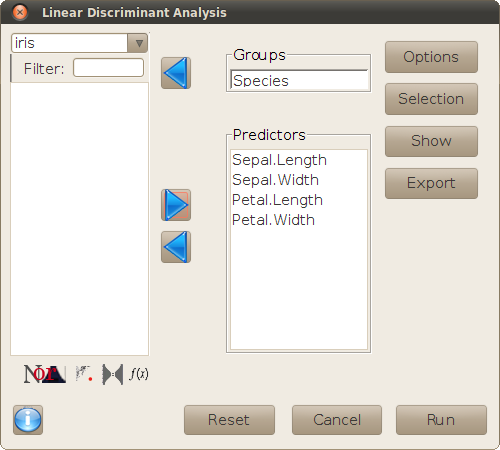The buttons on the right hand side of the dialog open new sub-dialogs, for setting the options of the analysis and performing additional operations, as explained in the following sections.

## Options

This sub-dialog sets the options of the `lda` function used for the analysis.

• Prior probabilites defines the initial classification criterion. It is possible to choose between 'Observed' probabilities (the probability of each category is proportional to its observed frequency), or 'Equal' probabilities (all categories have the same probability).
• Method defines the procedure for estimating mean and variance of the variables. It is possible to choose between 'Moment', 'MLE' (maximum-likelihood estimators), 'MVE' (minimum volume ellipsoid), or 'Robust (t)' (based on a t distribution). If the latter is chosen, the degrees of freedom of the distribution are set in the 'Df' edit field.
• Tolerance to singularity defines the minimum variance allowed for variables and linear combinations of variables.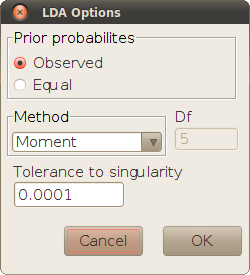## Selection

LDA is normally used when there is an educated inital hypothesis about the frequency of each category, but not necessarily about how those frequencies are related to the independent variables. Therefore, the initial selection of independent variables is often overredundant and includes variables that may be irrelevant for the classification.

The sub-dialog Selection allows to do a stepwise variable selection before the analysis, using the Wilks' lambda criterion, to keep only the independent variables that have a relatively important explanatory power. The selection starts with the variable that discriminates best between categories, and new variables are progressively added, while the gain in explained variance is over a threshold (indicated by a maximum p-value for the comparison between the current selection and the next step).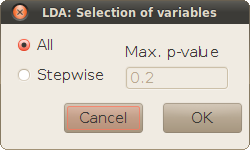This sub-dialog is used to print tables and plots that give additional information about the linear discriminant functions and the goodness of a posteriori classification.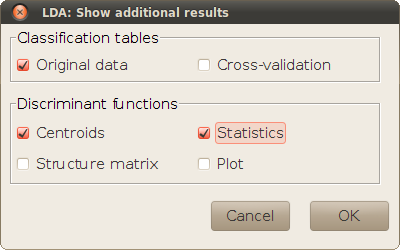### Classification tables

The classification tables show how many of the originally observed cases would be correctly reclassified (in the diagonal cells) or misclassified (off-diagonal cells), using the LDA model. The actual discriminant functions that are used for the reclassification depend on the selected checkboxes. If Original data is selected, the discriminant functions are calculated from the whole data set for all observations. With Cross-validation, a different set of discriminant functions is used for each observation, calculated by leaving out that observation (a Jacknife re-classification). The availability of Cross-validation tables depends on the method selected for the analysis in the Options sub-dialog.

### Analysis of discriminant functions

The checkboxes within this panel are used for showing detail analyses about the discriminant functions:

• Centroids: print the average values of the discriminant functions for each category of the observations. The distance between these 'centroids' indicate the ability of each function for separating categories.
• Statistics: prints two tables that analyze the discriminatory power of the different functions (that are ordered by decreasing discriminatory power):
• One-way ANOVA of each function with the categorical outcome as factor. This table is a test of significance of the distance between centroids in each function's scale.
• Wilks' lambda and Canonical correlation of each function. These statistics are usual descriptors of the discriminatory power, and are based on the proportion of trace of each function (printed in the standard output of `lda`). Wilks' lambda is a ratio of unexplained variance that may vary between 0 and 1, so that the lower is this value, the more a function discriminates between categories. The table shows a Wilks' lambda for each function, although this statistic is usually calculated for groups of functions as well, by multiplying the individual lambdas. For instance, the lambda for the two first discriminant functions is just the product of the first and second lambdas. The meaning of the 'canonical correlation' is just opposite to Wilks' lambda: it is equivalent to the root square of the R2 coefficient calculated in each ANOVA.
• Structure matrix: a matrix with the correlations between each discriminant function and the independent variables. It indicates the 'weight' of the original variables on the discriminant functions.
• Plot: plot the observed values of the discriminant functions for the different categories. The aspect of the plot depends on the number of categories: an histogram for binary classifications, and scatterplots for three or more categories. Such plots provide a visual representation of the results that are printed in the additional tables.

## Export results

The result of LDA is often used for classifying new cases where only the independent variables is known. This subdialog allows to save the result of this operation, and intermediate calculations if wanted (the values of the discriminant functions, and the a posteriori probabilities for each category whence the classification criterion comes). The data frame selected for this operation must have the same independent variables as the data frame used for defining the model. The same data frame is chosen by default. (Leaving that data frame would result in the reclassification used for calculating the first clssification table in the sub-dialog Show.)